# Force between two parallel wires

An interesting effect occurs if we consider two long straight parallel wires separated by a distance d carrying currents I1 and I2 . Let us examine the case where the two currents are in the same direction, as in Fig. 1.9.Wire #2 will experience a magnetic field of Eq.(1.6) due to wire #1 given by

 B1 =(10)

in the direction indicated, and hence will experience a force per unit length given by Eq.(1.4):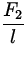=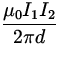. (11)

The direction of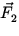indicated shows that wire #2 will be attracted towards wire #1. In a similar manner, one can show that wire #1 will experience a force due to the magnetic field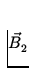of wire #2, and that this force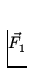will have a magnitude equal to that of F2 given in Eq.(1.11) but opposite in direction. Thus, wire #1 will be attracted towards wire #2.

It is a good exercise to show that if the wires were carrying currents in the opposite directions that the resulting forces will have the same magnitude as in Eq.(1.11) but are such as to cause a repulsion between the wires.

This force between two current carrying wires gives rise to the fundamental definition of the Ampère:

If two long parallel wires 1 m apart each carry a current of 1 A, then the force per unit length on each wire is 2 x 10- 7 N/m.
This definition of the Ampère then gives rise to the basic definition of the unit of charge, the Coulomb:
A wire carrying a current of 1 A transports past a given point 1 C of charge per second.
This definition also explains the reason why the constantof Eq.(1.7) was given exactly as 4x 10- 7 Tm/A.Next: Problems Up: Magnetism Previous: Solenoid